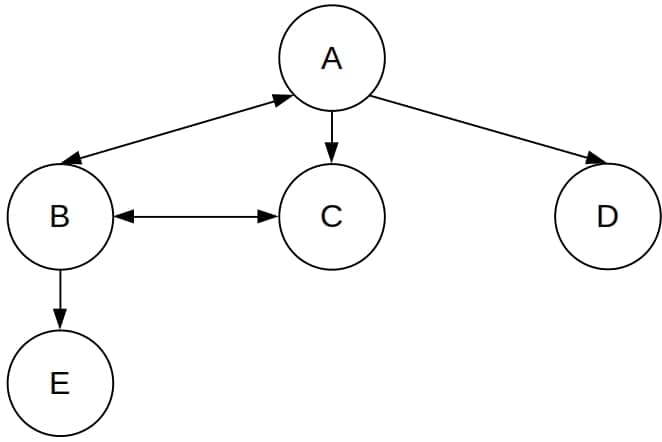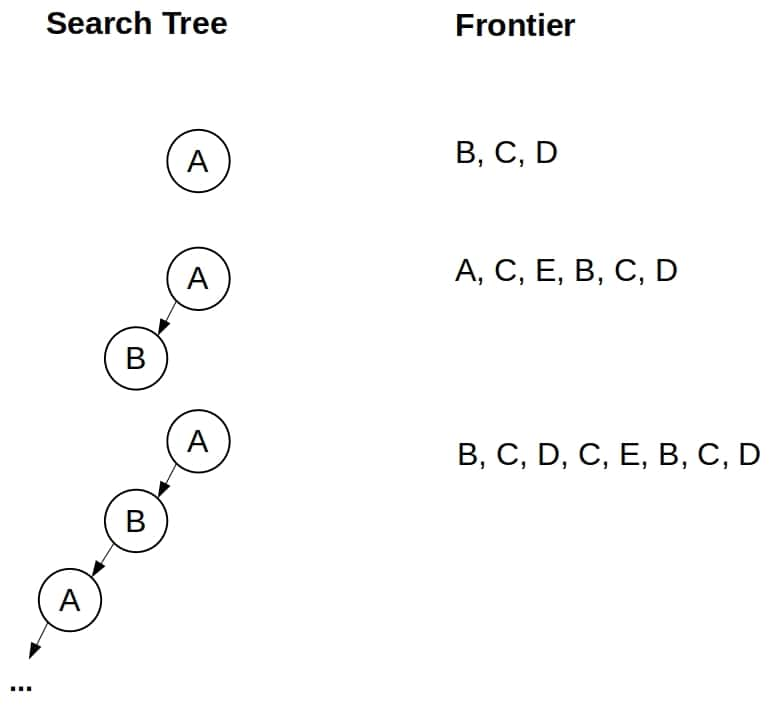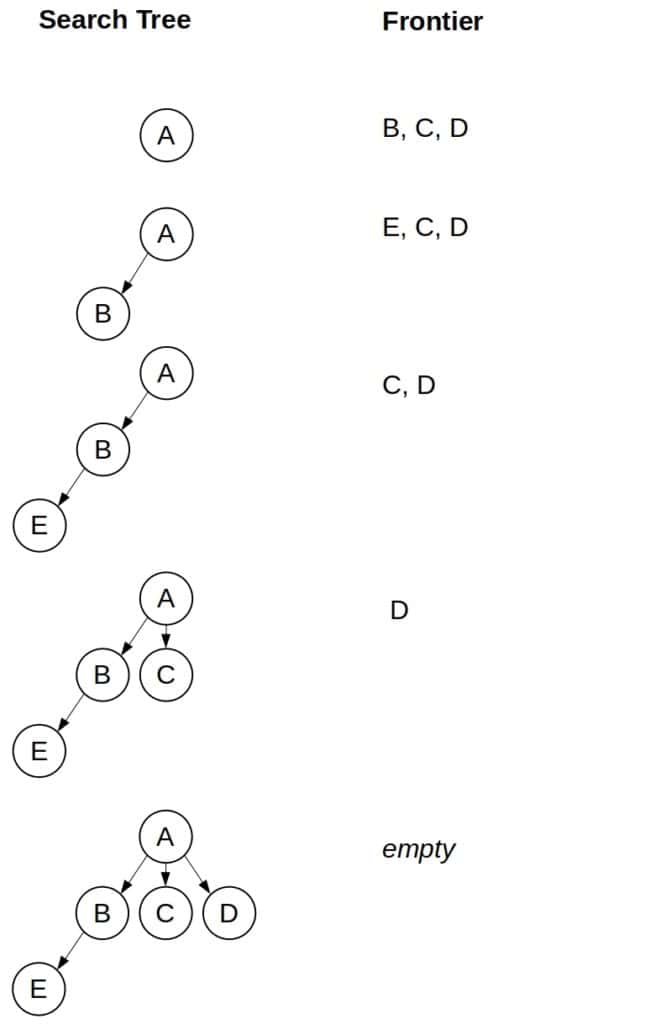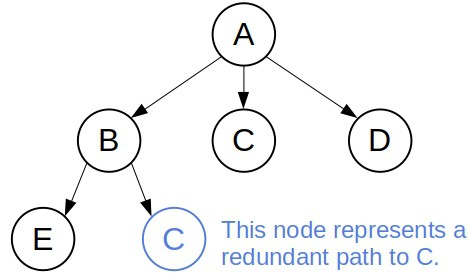## 1. Introduction

In this tutorial, we’ll talk about two techniques for solving search problems in AI: Graph Search (GS) and Tree-Like Search (TLS) strategies.

## 2. Search Problems and State Graphs

Search problems are those in which an AI agent aims to find the optimal sequence of actions that lead the agent from its start state to one of the goal states. All the possible states an agent can be in, along with the links that show which action transforms one state to another, constitute a state graph.

A state can be anything, depending on the problem: a point on a 2D map, the order in which to assemble the pieces of a product,  an arrangement of the chess pieces on the board, and so on.

## 3. Search Trees

Search algorithms differ by the order in which they visit (reach) the states in the state graph following the edges between them. For some algorithms, that order creates a tree superimposed over the state graph and whose root is the start state. We call that tree a search tree and will consider only the algorithms that grow it.

But, how do we grow a search tree in those algorithms? Whenever we reach a state, we create a node we mark with that state. Later, we insert the node into the tree as a child of the node from whose state we reached it.

We see the differences between the states in the state graph and nodes in the search tree. Each state appears in the graph only once. But, it may appear in the tree multiple times. That’s because, in the general case, there may be more than one path from the start state to any other state in the graph. So, different search-tree nodes marked with the same state represent different paths from the start to that state.

There lies the difference between the tree-like and the graph search strategies. The latter avoids repeating the states in the search tree.

### 3.1. Frontier

To see how the graph search strategy does that, we should first differentiate between reaching and expanding a state. We reach a state when we identify a path from the start state to it. But, we say that we expanded it if we had followed all its outward edges and reached all its children.

So, we can also think of a search as a sequence of expansions, and we first have to reach a state before expanding it. Therefore, we have to keep track of the reached but unexpanded states because we can expand only them. We call the set of such states the frontier. But, we have to be careful. Since there may be multiple paths to any state in general, we can reach a state more than once. Each time we do that, we get a new candidate node for the tree.

For those reasons, we conclude that a search strategy has two components:

• rule(s) to decide whether or not to place the node in the frontier
• rule(s) to choose the next frontier node for expansion

## 4. Graph Search (GS)

GS has one rule regarding the frontier:

Don’t add a node if its state has already been expanded or a node pointing to the same state is already in the frontier.

All the algorithms that conform to it belong to the class of graph-search methods. The generic pseudocode of GS is:We should note that there may be no algorithm-specific conditions for adding a node to. In that case,is a function that always returns.

### 4.1. Implementation Details

From Algorithm 1, we see that we need a special data structure for nodes. Since a node represents a path to its state, it has to include at least the pointer to the state’s predecessor on the path in question. So, a node should be an object with the following attributes:

• the state
• the pointer to the node’s parent: the node containing the state’s predecessor

In addition, we’d benefit from including these pieces of information as well:

• the action applied to jump from the parent to the node
• the total cost of the path

The way we implementdepends on the algorithm “subclassing” the generic form of GS. In UCS,is a min-priority queue, but in DFS, it’s a LIFO queue. The implementation ofshould be compatible with.

There’s also more than one way to implement. We can use a set or a key-value structure with states as keys and the corresponding nodes as values. Whatever we choose,should support fast look-ups and inserts (and deletions and updates in the cases like UCS).

We can also change the point at which we apply the goal test. Here, we do it after choosing a frontier node for expansion. That’s compatible with UCS. But, we can also test the nodes before adding them to the frontier. The latter approach wouldn’t work in UCS but will in DFS and BFS. Anyhow, the point at which we conduct the goal test doesn’t determine if an algorithm is of type GS or TLS. So, we can move the test to the inner for-loop of Algorithm 1 and still have the generic GS.

### 4.2. But, Where’s the Search Tree?

We introduced the GS and TLS strategies with the search trees they superimpose over the state graphs. However, there’s no reference to the tree in the generic form of GS. Why?

That’s because the search tree is implicit. We implicitly grow the tree each time we expand a node as we consider it has thus become the tree’s new leaf. So, the search tree in GS is made of the nodes that are present inbut not in.

## 5. Tree-Like Search (TLS)

We get the generic pseudocode of TLS by removing all the references tofrom the generic GS algorithm:All our remarks regarding the functionand the implementation details offor GS also hold for TLS. The same goes for the implicitness of the search tree.

Because TLS doesn’t check for repeated states, it may expand the same state multiple times. That can increase the running time and even lead to infinite loops if the state graph contains cycles.

## 6. Example

In this section, we’ll show how to implement the GS and TLS versions of DFS. We’ll also show those two versions of the same algorithm behave differently in practice. As the example, we’ll use the following graph:In it,is the start state, but no state is a goal.

### 6.1. Depth-First Search (DFS)

The idea behind Depth-First Search is to expand the node whose state we’ve reached the most recently. So, we use a LIFO queue as the frontier. We increase the depth of the search tree as much as we can until there are no more nodes to add or we reach a goal state.

In DFS, we usually run the goal test after reaching a state and not after choosing a node from the frontier. That way, we make the algorithm more efficient, but it isn’t incorrect to test the nodes after selecting them for expansion.

### 6.2. Tree-Like DFS

Here’s the pseudocode of TLS DFS:Let’s suppose thatreturns the nodes in alphabetical order. This is how TLS DFS would handle our example:As we see, TLS DFS gets stuck in a loop and runs indefinitely even though the state graph is finite and small.

### 6.3. Graph-Search DFS

We can get a GS DFS if we memorize all the states we’ve reached and test for repetitions:As we see, GS DFS avoids getting stuck in the loop:## 7. Discussion

Which strategy is better? It may seem that GS is always superior to TLS because it can’t get stuck in the loop as TLS can. However, the answer isn’t as straightforward as it may seem. GS can have large memory requirements since it has to memorize every single state it reaches.

Further, if the state graph is indeed a tree, so it doesn’t contain cycles, we should go with a TLS algorithm instead of GS. The latter would take unnecessary memory. Sincecan only grow, it may even become too big for RAM, taking a toll on the execution time due to trashing.

However, we can find a compromise between GS and TLS. In general, a loop is a special case of a redundant path. If the path represented by the nodeis longer or more costly than the one represented by node, we call the former path redundant. Provided that all actions have a non-negative cost, all the loops are redundant.

The compromise would consist in checking only for loops and not for other types of redundant paths. The only thing we need to add to TLS to get a loop-resistant algorithm is a check ifis somewhere on the path represented byin the inner for-loop.

For example, the loop-resistant DFS would avoid the cycle that TLS DFS gets stuck in but would still expandtwo times:## 8. Conclusion

In this article, we presented the Graph-Search and Tree-Like Search strategies. Even though the former avoids the loops, it is more memory-demanding than the latter. Which approach is appropriate depends on the search problem at hand.